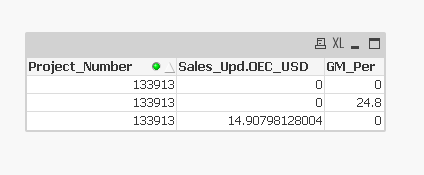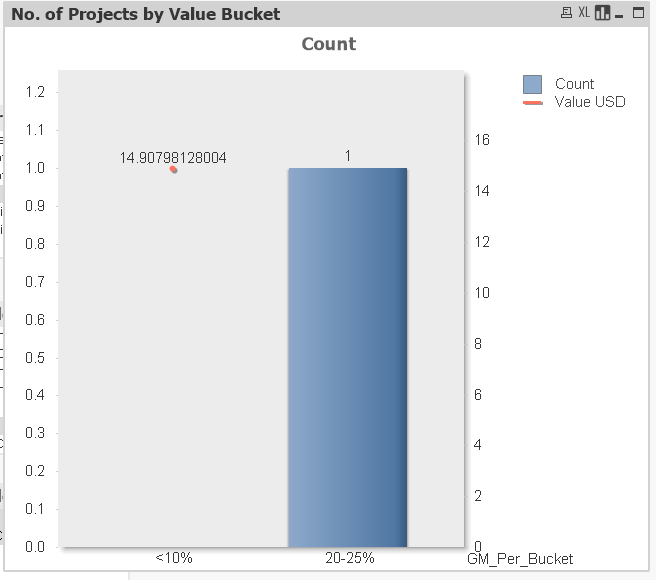# New to QlikView

Discussion board where members can get started with QlikView.

Announcements
Modernize Your QlikView Deployment webinar, Nov. 3rd. REGISTER
cancel
Showing results for
Did you mean:
HighlightedCreator III

## How to do Sum of the values per project?

Hello,

I have Project_number, Report Parameter and Value columns in my table. For every project, there are multiple Report Parameters like Sales, GM_Per, Risk and the values associated with them. Something as shown in below picture;

 Project_No Report_Param Value 113913 Sales 14.907 113913 GM_Per 24.80% 113913 Risk 21.25 123456 Sales 31.5 123456 GM_Per 11.20% 123456 Risk 9.87

Here is the table chart I am getting for project no.113913 as shown belowI am trying to get a visual like as shown below, Please help me to modify the script to get sum all the values and build a table like this.

So that I can compare the Sales vs Gross_Profit _Percentages.

Project_noSum(Sales)Sum(GM_Per)
13391314.90724.8

Any help is greatly appreciated

thanks

Bhavesh

10 Replies
HighlightedChampion III

May be try this?

Dim: Project_no

Expr1: Sum(TOTAL <Project_no> Sales)

Expr2: Sum(TOTAL <Project_no> GM_Per)

OR

= Sum(Aggr(Sum(Sales), Project_no))

= Sum(Aggr(Sum(Gm_Per), Project_no))

Oops did not realize you have field values for Sales and GM_Per. Try like below

Param:

* INLINE [

Project_No, Report_Param, Value

113913, Sales, 14.907

113913, GM_Per, 24.80%

113913, Risk, 21.25

123456, Sales, 31.5

123456, GM_Per, 11.20%

123456, Risk, 9.87

];

Then using Straight table add Project_No and expr as

Sum(Sales)

Sum(Gm_Per) * 100

HighlightedMVP

Use Project_No as dimension and as expressions

=Sum({<Report_Param = {'Sales'}>} Value)

=Sum({<Report_Param = {'GM_Per'}>} Value)

HighlightedCreator III

Hi, I am trying to build a Bar chart with Line Axis Comparing the GM_Per range vs Sales amount. as shown belowPlease tell me how to resolve this?

HighlightedSpecialist II

You can also use the Generic load as

tab1:

Generic

Project_No Report_Param Value

113913 Sales 14.907

113913 GM_Per 24.80%

113913 Risk 21.25

123456 Sales 31.5

123456 GM_Per 11.20%

123456 Risk 9.87

] (delimiter is '\t');

HighlightedCreator III

Hi, please look at my reply above I am trying to achieve Bar chart with Line axis.

HighlightedChampion III

is this a different question? You asked to see the output in a table? Am i missing something here? And where is this Bucket coming from in your sample posted intially?

HighlightedMVP
HighlightedCreator III

I have GM_Buckets in my script;

if(GM_Per >= 0.1 and GM_Per <= 10, '<10%',

if(GM_Per > 10 and GM_Per <= 14 ,'10-14%',

if(GM_Per > 14 and GM_Per <= 20, '14-20%',

if(GM_Per > 20 and GM_Per <= 25, '20-25%',

if(GM_Per > 25 and GM_Per <= 30, '25-30%',

if(GM_Per > 30 and GM_Per <= 35, '30-35%',

if(GM_Per > 35 and GM_Per <= 40, '35-40%',

if(GM_Per > 40, '>40%')))))))) as GM_Per_Bucket

I am using GM_Bucket as dimension and created the expression as sum({<Report_Param ={'Sales'}>}Value).

But When I try to create the Chart, this is what I am getting itHighlightedCreator III

I have GM_Buckets in my script;

if(GM_Per >= 0.1 and GM_Per <= 10, '<10%',

if(GM_Per > 10 and GM_Per <= 14 ,'10-14%',

if(GM_Per > 14 and GM_Per <= 20, '14-20%',

if(GM_Per > 20 and GM_Per <= 25, '20-25%',

if(GM_Per > 25 and GM_Per <= 30, '25-30%',

if(GM_Per > 30 and GM_Per <= 35, '30-35%',

if(GM_Per > 35 and GM_Per <= 40, '35-40%',

if(GM_Per > 40, '>40%')))))))) as GM_Per_Bucket

I am using GM_Bucket as dimension and created the expression as sum({<Report_Param ={'Sales'}>}Value).

But When I try to create the Chart, this is what I am getting it